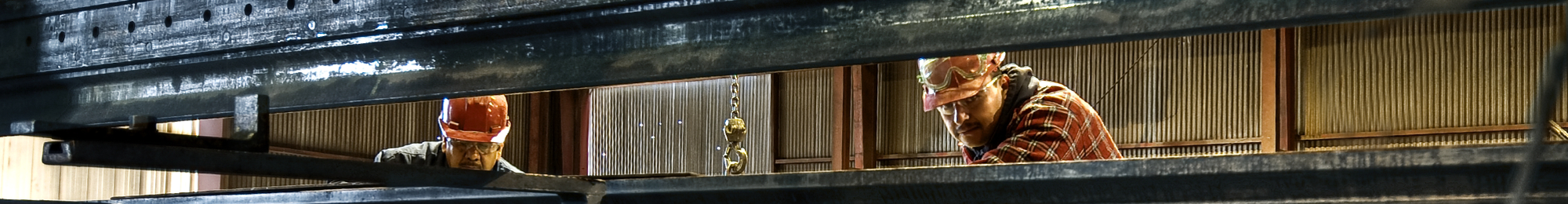Willbanks Metals# Metric Conversion Factors

Metric to English English to Metric
kilograms x 2.2046 = pounds pounds divided by 2.2046 = kilograms (kg)
kilograms divided by 907.2 = tons (2000 lbs.) tons (2000 lbs.) x 907.2 = kilograms (kg)
millimeters x 0.03937 = inches inches divided by 0.03937 = millimeters (mm)
or millimeters divided by 25.4 = inches or inches x 25.4 = millimeters (mm)
meters x 39.37 = inches inches divided by 39.37 = meters (m)
meters x 3.281 = feet feet divided by 3.281 = meters (m)
meters x 1.094 = yards yards divided by 1.094 = meters (m)
sq. millimeters x 0.00155 = sq. inches sq. inches divided by 0.00155 = sq. millimeters (mm/2nd power)
or sq. millimeters divided by 645.16 = sq. inches or sq. inches x 645.16 = sq. millimeters (mm/2nd power)
Metric to English English to Metric
WEIGHTS = 453.6 grams, or 0.4536 kilograms (kg)
1 pound (1 lb.) = 1.4882 kilograms per meter (kg/m)
1 lb. per foot (lb./ft.) = 907.18 kilograms (kg)
1 net ton (2000 lbs.) = 1016 kilograms, or 1.0160 metric tons
1 gross ton (2240 lbs.) = 305.15 grams, or 0.30515 kilograms per sq. meter (kg/m to the 2nd power)
1 ounce per sq. foot = 2.2046 lbs.
1 kilogram (kg) = 0.67197 lbs. per foot
1 kilogram per meter = 2204.6 lbs.
= 1.10231 net tons
1 metric ton (1000 kg) = 0.98421 gross ton
MEASURES
1 inch (in.) = 25.40 millimeters (mm)
1 sq. inch (in./to 2nd power) = 645.16 sq. millimeters
1 sq. foot (ft/to 2nd power) = 0.092903 sq. meter
1 millimeter (mm) = 0.03937 inch
1 sq. millimeter (mm/to 2nd power) = 0.00155 sq. inch
1 meter (m) = 3.2808 feet
= 1.09361 yards
1 sq. meter (m/to 2nd power) = 10.7639 sq. feet
= 1.196 sq. yards
TEMPERATURE
Celsius (formerly called the centigrade scale)
tc = temperature (Celsius) in degrees
tf = temperature (Fahrenheit) in degrees
tc (tf – 32)
1.8